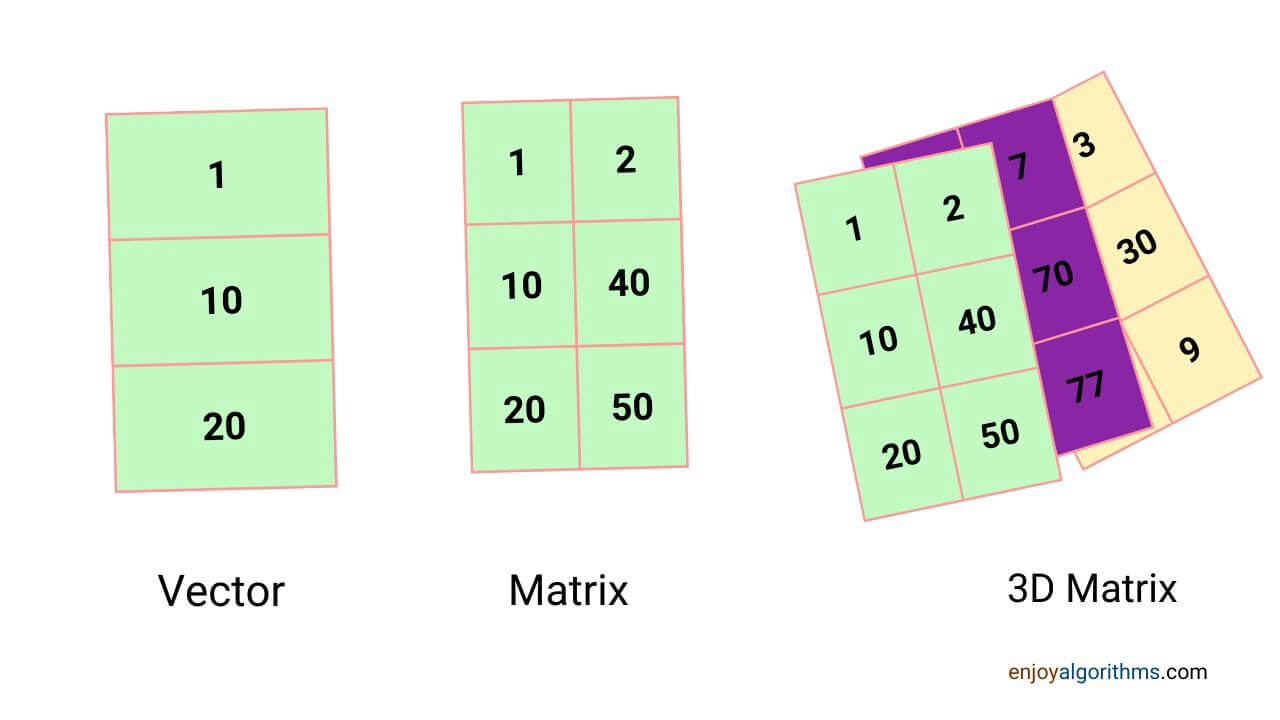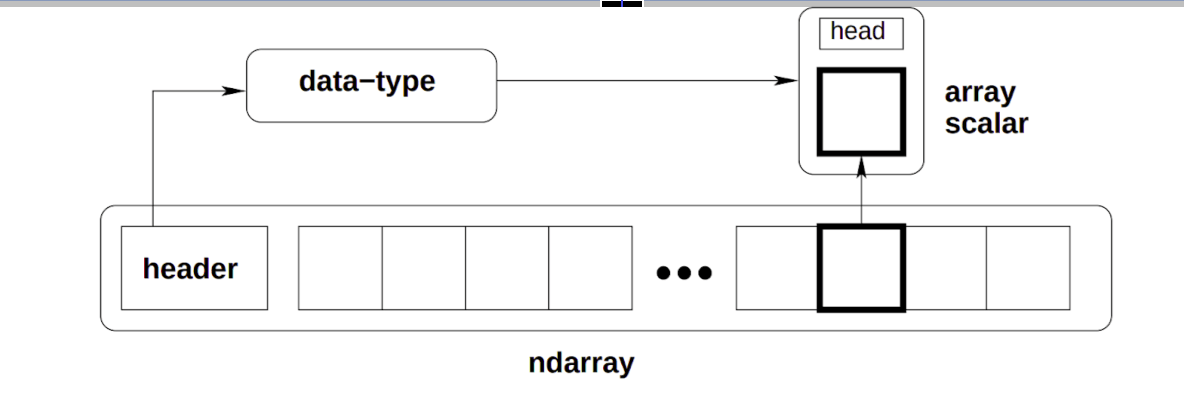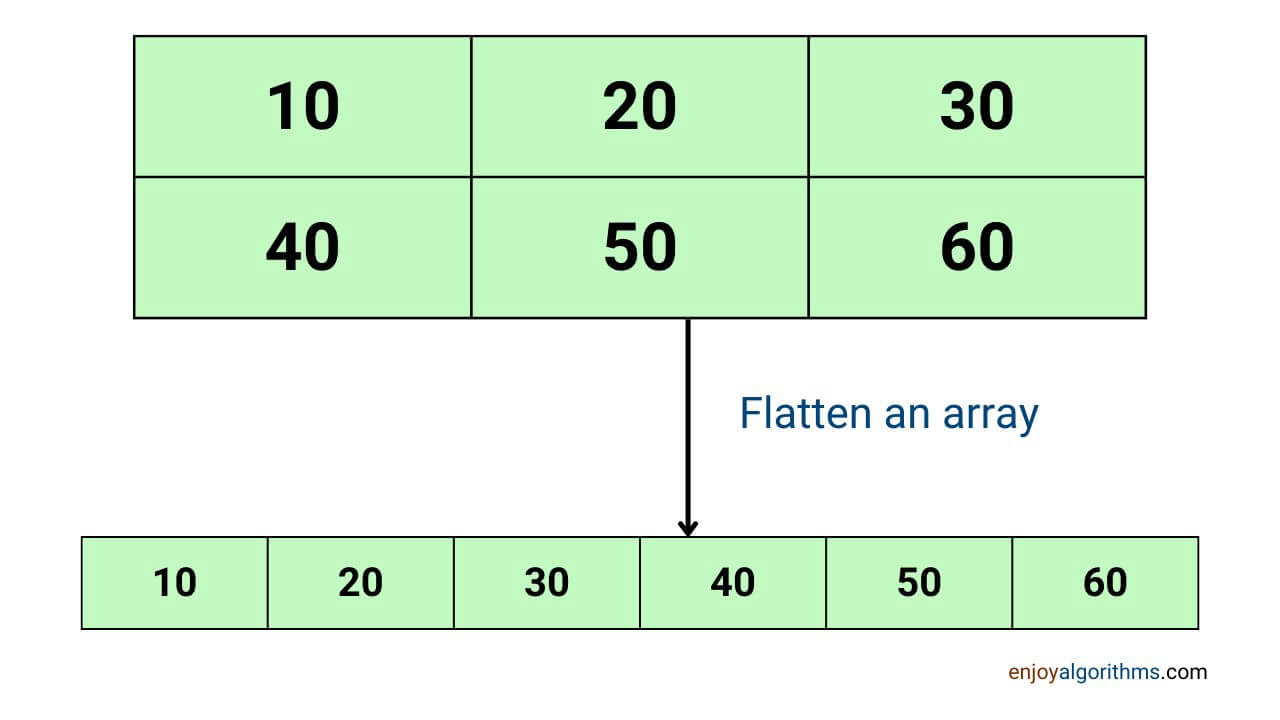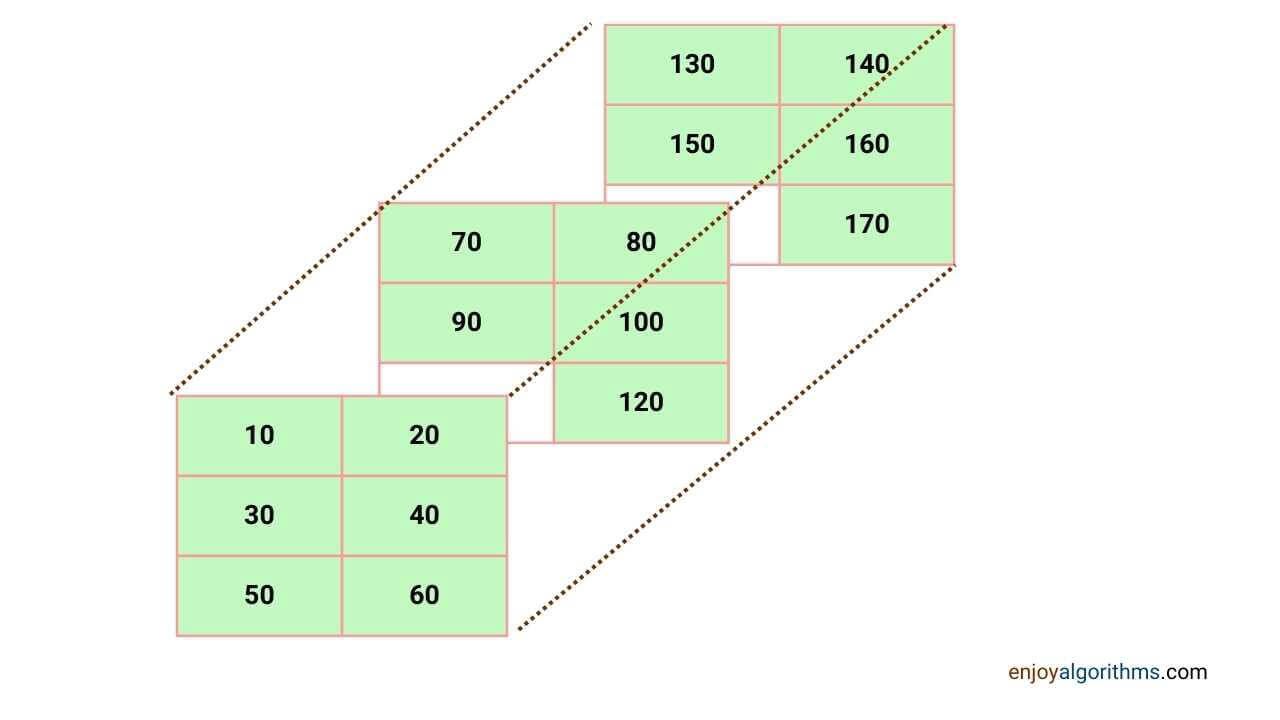# Introduction to NumPy Library in Python

Numerical data is the most commonly used data type in Machine Learning and Data Science. On the other side, handling large amounts of numerical calculations (which can reach billions) requires more than just standard Python methods. This is where NumPy, a popular Python library, steps in to provide efficient storage and mathematical operations.

NumPy is a core package that is used in almost all machine learning projects, making it an essential skill for data professionals to master. Therefore, the objective of this blog is to cover frequently used NumPy functions and guide you through the installation process.

### Key takeaways from this blog

• What is NumPy, and how is it different from Python lists?
• How can we create a NumPy array?
• The installation process of NumPy
• Important operations like finding the shape, reshaping, squeezing, expanding, slicing, indexing, concatenating, stacking, broadcasting, and some essential mathematical operations.
• Use cases of Numpy in machine learning

Let us start by learning more about NumPy.

### What is NumPy?

In 2005, Travis Oliphant released an open-source library to perform mathematical operations on large multidimensional arrays efficiently and named it NumPy. Because of its effectiveness, it became the core library and building block of other important Python libraries, such as Pandas, Matplotlib, Seaborn, and Scikit-learn.

### What is a Numpy Array?

NumPy allows us to store numerical values in single or multiple dimensions using NumPy arrays. Unlike Python arrays and lists, NumPy arrays are a unique structure which contains partial properties of both of them. Generally, a one-dimensional array is known as a vector, a two-dimensional array is a matrix, and an array with three dimensions is called a tensor (a set of matrices). NumPy arrays are given the unique name of N-dimensional arrays or ndarrays.One critical question is: How are ndarrays different from Python lists or inbuilt Python arrays?

#### Difference between Lists, Python arrays, and Numpy arrays

Python lists are versatile and can store different data types in a single structure, but they are not efficient when it comes to memory usage and computing efficiency. Each cell in a list must store information about the type of object it holds, which can lead to significant memory overhead for large datasets.

NumPy arrays, on the other hand, are designed to store elements of similar data types and store general information about the data type at the start. This makes NumPy arrays an ideal choice when working with large datasets that require efficient memory usage and computation.Python's built-in arrays are rigid when it comes to data types i.e. you cannot store elements of different data types in the same array. If you attempt to store a float value in an array defined for int values, you will get an error. In contrast, NumPy arrays are much more flexible in terms of data types. They can automatically convert data types to ensure homogeneity i.e. if you pass float values to an int-typed NumPy array, the float values will be automatically converted to int and stored.

In NumPy arrays, the data type is stored in the header, this helps us in efficient access to the data. When a specific element of an array is indexed, the value is retrieved from the array, and the data type is retrieved from the header. This efficient access to the data makes NumPy arrays an ideal data structure for handling large amounts of numerical data, which is often the case in machine learning and data science applications.

• NumPy makes element-wise operations possible, which is impossible with lists.
• NumPy breaks tasks into multiple fragments and executes them in parallel.
• NumPy is also time-efficient for mathematical operations. This is due to parallel computing and the base code of NumPy being partly in C, C++, and Fortran, which gives it a faster execution time.

We have seen the difference. Let us see some practical use cases of NumPy.

### Use Cases of Numpy in Machine Learning

We perform mathematical analysis and calculations such as finding the mean, median, and variance of data samples, applying filters on features, performing matrix multiplication, or finding gradients. All these calculations can be done within Python, but NumPy makes them 50 times faster. It makes Numpy the first choice for development.

Some good use cases of Numpy are:

• Numpy provides the functionality of generating random numbers, which can be used to initialize the parameters for machine learning and deep learning models. These random numbers can follow a particular probability distribution per our needs. For example, it can give us numbers following a normal distribution.
• Numpy is used to apply smoothening operations on data features, making the training of machine learning models stable and efficient.
• Numpy is used to calculate the gradient of vectors to update the parameters while iterating over the training dataset. This is used in the core part of learning while training ML models.
• Numpy is used to perform huge matrix multiplication. The number of parameters can range in billions, and performing matrix multiplication on them can be a massive bottleneck while training DL models, but Numpy handles it very efficiently.

It holds much more potential than what we mentioned till now, making it an integral and essential library to learn about. So let's begin with the installation and know some essential supports it provides.

### Installation and Import of NumPy

One can find the detailed instruction to install NumPy on all operating systems in our make your system machine learning-enabled blog. To install NumPy via Python PyPI (pip), we can use the commands below:

``````Python2 on terminal → pip install numpy
Python3 on terminal → pip3 install numpy
Jupyter notebook python2 → !pip install numpy``````

Once installed, we can import this library and use it in our codes. For example:

`i﻿mport numpy as np`

The Numpy library is imported with a new name of "np". So in future sections, whenever we call 'np', it will indirectly refer to Numpy. Let's first learn about creating a numpy array using the numpy library, and then we will see its mathematical operations.

### Creating a NumPy Array

#### Converting lists into a numpy array

We can convert a list, a native data structure in Python, into a numpy array using the np.array() function. For example:

``````np.array([1,2,3])

#Output:
array([1, 2, 3])``````

We can also specify the data type inside the "np.array" function. Suppose we select a data type as "int", but the input list has float values. In that case, while creating an array, it will take the floors of those float values, as shown in the example below. Please note the difference between "dtype" and the corresponding output.

``````np.array([1,2,3.7],dtype = int)

Output:
array([1, 2, 3])

np.array([1,2,3.7],dtype = float)

Output:
array([1., 2., 3.7])``````

As discussed earlier, a NumPy array can be multidimensional, and the same can be created by passing a list of lists to the np.array() function. For example, a 2x3 NumPy array can be created as follows:

``````np.array([[1,2,3],[4,5,6]])

#Output:
array([[1, 2, 3],
[4, 5, 6]])``````

#### Array with a fixed number

The np.full() function can create an array containing a fixed number. We provide the array's shape and the number we want to fill in that array. This method is useful while assigning the same value to all the parameters during the training of a machine learning model. Let's take a look at an example.

``````np.full((2,2),5) # Shape is 2X2 and we want to fill 5 in this array

Output:
array([[5, 5],
[5, 5]])``````

There is one extra function, np.zeros(), which creates an array with all elements zero. We need to pass the shape of the array as a tuple to this function, and it will provide the array. For example:

``````np.zeros((2,2))

#Output:
array([[0., 0.],
[0., 0.]])``````

Similarly, np.ones() will give us the array of required shapes with all elements 1. For example:

``````np.ones(4)
# Output:
array([1., 1., 1., 1.])``````

#### Random array generation using Numpy

In most Machine Learning applications, random values are assigned to the parameters, which are then fine-tuned based on training samples. The NumPy function np.random.rand() is used to create an array with random values. These random values lie in the range of [0, 1), including zero but excluding 1.

``````np.random.rand(2,3)

#Output:
array([[0.76981844, 0.56005659, 0.61075499],
[0.2434684 , 0.8560164 , 0.22834211]])``````

#### Identity Matrix Creation using Numpy

An identity matrix is a square matrix in which only diagonal elements are one, and the rest are zero. These matrices are very useful while constructing the deep-learning architecture and can be created using the np.eye() function. It expects the input argument to represent the number of rows for an identity matrix to create. Since an identity matrix is square, the number of columns will be equal to the number of rows. For example:

``````np.eye(4)
# Output:
array([[1., 0., 0., 0.],
[0., 1., 0., 0.],
[0., 0., 1., 0.],
[0., 0., 0., 1.]])``````

We can shift the diagonal of an identity matrix upward or downward by specifying the value of k in the np.eye(number of rows, k=value) function. If the value is positive, it shifts the diagonal upward; for a negative value, it shifts the diagonal downward. Please note that the resulting matrices obtained with a non-zero value of k are not identity matrices. An example is shown below where the diagonal is shifted downward:

``````np.eye(4,k=-1)
# Output:
array([[0., 0., 0., 0.],
[1., 0., 0., 0.],
[0., 1., 0., 0.],
[0., 0., 1., 0.]])``````

#### Array elements with a constant distance

Arrays in which the difference between consecutive elements remains constant are known as evenly-spaced arrays. We can use the np.arange() method to get an evenly spaced array like this:

``````np.arange(0,10,3) ## np.arange(start, end, gap)

Output:
array([0, 3, 6, 9])

np.arange(4) ## The default gap is 1 and start is 0

Output:
array([0, 1, 2, 3])``````
``````#np.arange(starting_point, end_point, step_size)
np.arange(10,30,5)
Output:
array([10, 15, 20, 25])``````

In the above example, please note that the endpoint is not included in our array. So 30 is not included in the array as it was our endpoint. If we want the endpoint to be included, there is an alternate function called np.linspace(). Here, we specify the number of elements we want in the array instead of the step size, as shown:

``````#np.linspace(starting_point, end_point, number_of_elements)
np.linspace(10,30,6)
Output:
array([10., 14., 18., 22., 26., 30.])``````

We have learned to make a new array with the help of different methods. Let's see how to find the shape of an already existing ndarray.

### Different Dimensions of ndarray

We need to know the array's number of rows, columns, and axes to get an idea about the shape and size of the array. Let's create an array with the name np_array, which will be directly used for explaining different functions ahead, as shown:

``np_array = np.array([[10,20,30],[40,50,60]])``
``````10    20     30
40    50     60

Shape: (2,3)  Size: 6   N-Dim: 2``````

#### Number of axes of the array

We can use the ndim attribute to get the number of axes (also known as dimensions) of an array, as shown:

``````np_array.ndim

Output:
2

# We got an output as 2 as the array has two axes.``````

If the array contains three dimensions, then the value will be 3.

#### The shape of the array

Ndarrays support the shape attribute to get the shape of an array. It returns the result in a tuple, which indicates the number of entries in each dimension of a ndarray. For example, the output (2,3) states 2 types of entries on axis 0 and 3 types of entries for axis = 1.

``````np_array.shape
# Output:
(2,3)``````

We can also get the size of the array, which is the multiplication of each type of axes. For that, numpy provides the size attribute. For the example, we created, the output is 6 as axis1*axis2 = 2*3 = 6.

``````np_array.size
# Output:
6``````

#### Reshaping of ndarray

Sometimes we need to re-orient the existing elements in an array without changing the values of elements, and reshaping helps us with that. Reshaping becomes an important operation when we need to multiply two matrices, but their dimensions are not suitable. Let's take an example shown below.

``````a = np.array([10,20,30,40,50,60])
print(a)
a.reshape(2,3)

Output:
array([10, 20, 30, 40, 50, 60])
###below is the output after reshaping we get
array([[10, 20, 30],
[40, 50, 60]])``````

The input provided is the shape of the matrix we want. Please note that the matrix's size (multiplication of the entries of the shape) should be the same as the number of elements in the original array; otherwise, an error will occur.

``````## Error in reshaping to 2*5
Traceback (most recent call last):
File "<stdin>", line 1, in <module>
ValueError: cannot reshape array of size 6 into shape (2,5)

# The array a was having 6 elements which can not be filled in 2*5=10 places``````

In the above examples, we were aware of the whole shape of the required matrix. But sometimes, we know the one axis value and need to reshape it according to that. For that, we can give input as -1 in place of the dimension of the unknown axis.

``````a.reshape(3,-1)
Output:
array([[10, 20],
[30, 40],
[50, 60]])

a.reshape(-1,3)
Output:
array([[10, 20, 30],
[40, 50, 60]])``````

#### Transpose of a matrix using Numpy Library

Transpose is a shaping method where the number of rows and columns is swapped. For example,

``````np_array.transpose()

Output:
array([[10, 40],
[20, 50],
[30, 60]])``````

#### Flattening numpy array

We use the 'flatten' attribute to convert a multidimensional array to a one-dimensional array. A common use case for flattening can be merging multiple features before compression using PCA or auto encoder. We can use flatten() or ravel() for the same.

``````array1 = np_array.flatten()
array2 = np_array.ravel()
print("array shape after flatten is:",array1.shape)
print("array shape after ravel is:",array2.shape)
print("array after flatten is:",array1)
print("array after ravel is:",array1)
Output:
array shape after flatten is: (6,)
array shape after ravel is: (6,)
array after flatten is: [10 20 30 40 50 60]
array after ravel is: [10 20 30 40 50 60]``````flatten() returns a deep copy while ravel() returns a shallow copy. A deep copy creates an entirely new ndarray, and changes made to the output will not reflect in the original array. While in shallow copy, it refers to the original memory, which means that changes made to shallow copy output will also reflect in the original array.

``````###below is changes made in flatten output
array1 = 0
print(np_array)
Output:
[[10 20 30]
[40 50 60]]

###below is changes made in ravel output
array2 = 0
print(np_array)
Output:Output:
[[10  0 30]
[40 50 60]]``````

#### Expanding a NumPy array

We can use the np.expand_dims() method to extend the dimension of a numpy array. The input we need to provide is the array and axis along which we wish to expand the array. If the expansion is around rows, it will look like this:

``````np.expand_dims(a,axis=1)
Output:
array([,
,
,
,
])``````

#### Squeezing a NumPy array

Use the np.squeeze() method for compressing an array. Squeezing an array means reducing its dimension along an axis. The axis we choose has a corresponding value equal to 1 in the shape tuple. If, by chance, while selecting an axis, the condition of the corresponding shape value =1 is not followed, an error will occur.

``````a = np.array([[[1,2,3],[4,5,6]]])
a.shape
# Output:
(1, 2, 3)

np.squeeze(a,axis=2)
# Output: We get the following error as corresponding value is 3
Traceback (most recent call last):
File "<stdin>", line 1, in <module>
File "<__array_function__ internals>", line 180, in squeeze
File "/home/avisouser/.local/lib/python3.8/site-packages/numpy/core/fromnumeric.py", line 1545, in squeeze
return squeeze(axis=axis)
ValueError: cannot select an axis to squeeze out which has size not equal to one

np.squeeze(a,axis=0)
#Output:
array([[1, 2, 3],
[4, 5, 6]])``````

We have seen now to create an array and determine its shape. Now we will see how to access specific elements of an array using slicing and indexing.

### Slicing and Indexing of NumPy arrays

#### Slicing vectors or 1D arrays

Sometimes only a part of the complete array is needed. For that, we only need to pass starting index, end index, and step size as parameters in this order: [Start Index : End Index : Step Size]. For example, we have an array of sorted elements in ascending order, and we want to get all elements apart from the largest and the smallest element, then,

``````np.array([1,2,3,4,5,6])
np.array([1,2,3,4,5,6])
array([1, 2, 3, 4, 5, 6])``````
``````np.array([1,2,3,4,5,6])[1:5]
Output when no step size is: ## Default step size would be 1
array([2, 3, 4, 5])``````

Please note that here end index is not included. Step size determines the number of elements to skip from the array in choosing the next element. For example,

``````np.array([1,2,3,4,5,6])[1:5:2]
Output when step size is 2:
array([2, 4])  # Here note that element 3 is skipped``````

#### Slicing and indexing of matrices or 2D arrays

In 2D arrays, two axes are present. So slicing here has to occur for both axes. Please note that this method will also work for multidimensional arrays. Indexing elements in a 2D array is the same as we do indexing in the list of lists. For example,

``````###Indexing
np_array[0,0]
# Output: Here we get the elemnet from first row and first column
10

np_array[0,2]
# Output: Here we get the elemnet from first row and third column
30

np_array[1,2]
# Output: Here we get the elemnet from second row and third column
60``````

Let's see how we can do the slicing in the case of 2D arrays.

In the example below, slicing of ndarray along a column is performed, and all rows are chosen. Programmatically it can be done as:

``````###Slicing
np_array[:,1:2]

Output: Here we only choose the second column becuase start index is 1 and end index is 2, but 2 is excluded
array([,
])

np_array[:1,:]
Output: Here we only choose the first row
array([[10, 20, 30]])

np_array[:1,1:2]
Output: Here we only choose the first row and second column
array([])``````

#### Slicing and indexing of 3D matrices or 3D arrays

Let's create a 3D matrix using the np.array() method and then perform slicing,

``````a = np.array([[[10,20],[30,40],[50,60]],# first axis array

[[70,80],[90,100],[110,120]],# second axis array
[[130,140],[150,160],[170,180]]])# third axis array
print(a)

# Output:
[[[ 10  20]
[ 30  40]
[ 50  60]]
[[ 70  80]
[ 90 100]
[110 120]]
[[130 140]
[150 160]
[170 180]]]``````

Please note that the 3D matrix has an additional axis compared to the 2D matrix. The third axis determines the number of 2D matrices superimposed on one another, as shown in the figure below. So while slicing the 3D matrix, we need to mention which 2D array we want to slice.As discussed in the section Slicing and indexing of matrices or 2D arrays,we take slices of each axis to get our required elements.

``````a.shape
#Output:
(3, 3, 2)

## above we see that we get a 3d matrix with a depth of 2 and x, y axis as 3.

###Inexing of array
a[0,0,1]
#Output: Here we get first element for depth 1 with x and y coordinate being 0
20

###Slicing of array
a[1:,0:2,0:2]
# Output: We select first two rows of second and third array
array([[[ 70,  80],
[ 90, 100]],
[[130, 140],
[150, 160]]])``````

#### Flipping an array using Numpy

We can use the np.flip() method to flip the array horizontally or vertically, depending on the axis.

``````np_array
#Output:
array([[10, 20, 30],
[40, 50, 60]])

np.flip(np_array,axis=0)
# Output:
array([[40, 50, 60],
[10, 20, 30]])``````

### Stacking and Concatenating NumPy arrays

There are two ways to combine two ndarrays, Stacking and Concatenating. In stacking, the number of dimensions of the output array is more than the dimension of the input array, while in concatenation, it remains the same. For example, if we stack two 1-D arrays, we get a 2-D array, while concatenation will give a 1-D array only. In stacking, the axis along which arrays are combined should have the same size; otherwise, an error will occur.

We can use these functions for stacking and concatenation:

• Vertical stack (np.vstack()): Here, two arrays are combined vertically, increasing the number of rows.
• Horizontal stack (np.hstack()): Here, two arrays are combined horizontally, increasing the number of columns.
• np.dstack(): Here, two arrays are combined along depth and increase the array's depth.
• np.concatenate(): Here, we combine two arrays along a particular axis.
``````a = np.array([1,2,3])
b = np.array([4,5,6])
a1 = np.array([[10,20],[30,40]])
b1 = np.array([[50,60],[70,80]])
np.vstack((a,b))

# Output:
array([[1, 2, 3],
[4, 5, 6]])

np.hstack((a,b))
#Output:
array([1, 2, 3, 4, 5, 6])

np.dstack((a1,b1))
# Output:
array([[[10, 50],
[20, 60]],
[[30, 70],
[40, 80]]])

np.concatenate((a,b),axis=0)
# Output: Here we concatenate along row
array([1, 2, 3, 4, 5, 6])``````

Using broadcasting, we can apply simple arithmetic operations (addition, subtraction, etc.) on numpy arrays with different shapes. It beautifully leverages the functional property of Python and internally shifts some operations into a C environment rather than using Python, making execution faster.

It becomes beneficial in two cases:

• Multiplying a scalar with a ndarray.
• Increase the size of smaller ndarray for a case when two ndarrays have different dimensions. Note that the dimension, which does not match, has to be 1 for a smaller matrix, and then broadcasting would work. Otherwise, it will throw an error. For example, (5,6) ndarray can be broadcasted with (6,1).
``````a = np.arange(10,100,20)
b = np.array([,])

a+b
#Output: Here we get the output when we try to add 2 different dimensional ndarrays.
array([[13, 33, 53, 73, 93],
[13, 33, 53, 73, 93]])

a*2
# Output: Here we multiply by a scalar number for the whole matrix
array([ 20,  60, 100, 140, 180])``````

Here the scalar number is hypothetically stretched to match the dimensions of ndarray so that it becomes feasible for multiplication. Unless two ndarrays have the same dimensions, their calculations would not have been feasible, but now it is possible due to broadcasting.

### Mathematical Operations on NumPy array

#### Basic Arithmetic Operations

In standard mathematics, we apply addition, subtraction, division, etc. All this can be done for a Numpy array as well.

``````a = np.arange(10,100,20)
a
print("sum output is:",a+2)
print("subtraction output is:",a-2)
print("division output is:",a/2)

#Output:
array([10, 30, 50, 70, 90])
sum output is: [12 32 52 72 92]
subtraction output is: [ 8 28 48 68 88]
division output is: [ 5. 15. 25. 35. 45.]``````

Mean: We can find the mean of the values present in an array using the np.mean() method. For a vector, it means taking the sum of the vector and dividing it by the length of the vector.

Median: We can find the median value of an array using the np.median () method. The median is a value that separates the higher half from the lower half of data, a population, or a probability distribution.

Standard deviation: We can find the standard deviation using np.std(). Using standard deviation, we can find how much the data samples are dispersed with respect to the mean.

``````np.mean(a)
50.0

np.median(a)
50.0

np.std(a)
28.284271247461902``````

Minimum: We can find the minimum element in the array using the np.min() method. The index of a minimum element can be determined using the argmin() method.

Maximum: We find the max element in the array usingthe np.max() method. The index of the maximum element can be determined using the argmax()method.

Array Sum: We can usethe sum() method to find the array sum.

``````np_array.sum()
# Output:
210

np.min(a,axis=0)
# Output:
10

np.max(a,axis=0)
# Output:
90
### In above case we determine min and max element along the column``````

#### Sorting NumPy array

Often in Data Science problems, we need to sort elements. Depending on its implementation and algorithm used, the time required for sorting can vary greatly. NumPy provides inbuilt support for various algorithms like mergesort, quicksort, time sort, etc.

``````a = np.array([10,40,20,500])
np.sort(a, kind='mergesort')
# Output:
array([10, 20, 400, 5000])``````

### Conclusion

NumPy is a game-changer for Python developers as it enables efficient mathematical operations. This article covers the fundamentals of the NumPy library, including installation and working with ndarrays. For a more in-depth understanding, refer to the official documentation. We hope you found it informative and enjoyable.

References: https://numpy.org/doc/stable/

Enjoy Learning!

☆ 16-Week Live DSA Course
☆ 10-Week Live DSA Course